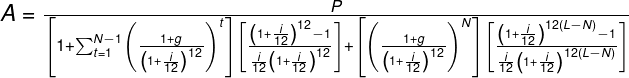# Graduated Payment Mortgage (GPM) Calculator

This graduated payment mortgage (GPM) calculator can be employed for the computation of the first and following payments required on a GPM for a variety of mortgage terms.

## What are Graduated Payment Mortgages (GPMs)?

A graduated payment mortgage (GPM) is a form of fixed-rate mortgage which begins with a low payment rate which gradually rises until a larger amount is being paid in the final stages. The monthly payments will begin low and see a gradual increase each year for between two and five years (or ten years, depending on the plan chosen) until they reach an agreed limit.

As an example, imagine you accept a graduated mortgage in which the monthly payments to begin with are \$960 with two annual "graduations" at 5% each. In the first year of your mortgage you would pay \$960 a month, in the second year \$1008 a month, and then for the third and subsequent years you would pay \$1058.40 a month. These mortgages can be useful to people who anticipate that their income will rise as the years go on.

## GPM Formula and Calculation Example

The formula below can be employed for calculation of monthly payment amounts for the mortgage's first year:Where:

A = monthly payment amount,

P = principal amount,

L = loan term in years,

N = number of graduations in GPM,

i = annual interest rate, expressed as a decimal,

g = graduated growth rate, expressed as a decimal.

To discover what the monthly payment will be in the second year, we multiply the payment in the first year by a factor of (1 + g). For calculating monthly payments in the third year, we simply do the same process with the second year payment.

Below is an example calculation to show how GPM functions:

Imagine you assume a loan of \$150,000 for 30 years, with the annual rate being 9%. You agree to graduated payment increases each year for the first five years and then a set steady payment after that, with increased rates of 7%. This gives us variables of P = 150,000, i = 0.09, M = 30, N = 5 and g = 0.07. If we put these values into our graduated payment formula we arrive at:

A = 150000 / [(4.787)(11.4341) + (0.8958)(119.2682)]

= 150000 / 161.5754

= 928.36.

In the initial year, there will be a monthly payment of \$928.36. To calculate what the payments will be in the following years, we just multiply the figure for the previous year by 1.07 up to our sixth year.

Year 1: \$928.36

Year 2: (\$928.36)(1.07) = \$993.34

Year 3: (\$993.34)(1.07) = \$1062.87

Year 4: (\$1062.87)(1.07) = \$1137.28

Year 5: (\$1137.28)(1.07) = \$1216.89

Years 6-30: (\$1216.89)(1.07) = \$1302.07

This compares with a 30 year fixed rate mortgage at 9% for which monthly payments from the start would be \$1206.93 a month.

Reference: Goebel, P.R. and N.G. Miller, 1981, Handbook of Mortgage Mathematics & Financial Tables, Englewood Cliffs, N.J.: Prentice-Hall.

You may also be interested in our Mortgage Overpayment Calculator or HELOC Payment Calculator